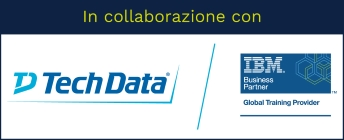Stai utilizzando un browser vecchio, che non rispetta gli standard web. Ti consigliamo di cambiarlo, perché questo sito potrebbe non funzionare correttamente.

# Introduction to Machine Learning Models Using IBM SPSS Modeler (V18.2)

## IBM0A079G

This course provides an introduction to supervised models, unsupervised models, and association models. This is an application-oriented course and examples include predicting whether customers cancel their subscription, predicting property values, segment customers based on usage, and market basket analysis.

### Argomenti

Introduction to machine learning models

• Taxonomy of machine learning models
• Identify measurement levels
• Taxonomy of supervised models
• Build and apply models in IBM SPSS Modeler

Supervised models: Decision trees - CHAID

• CHAID basics for categorical targets
• Include categorical and continuous predictors
• CHAID basics for continuous targets
• Treatment of missing values

Supervised models: Decision trees - C&R Tree

• C&R Tree basics for categorical targets
• Include categorical and continuous predictors
• C&R Tree basics for continuous targets
• Treatment of missing values

Evaluation measures for supervised models

• Evaluation measures for categorical targets
• Evaluation measures for continuous targets

Supervised models: Statistical models for continuous targets - Linear regression

• Linear regression basics
• Include categorical predictors
• Treatment of missing values

Supervised models: Statistical models for categorical targets - Logistic regression

• Logistic regression basics
• Include categorical predictors
• Treatment of missing values

Supervised models: Black box models - Neural networks

• Neural network basics
• Include categorical and continuous predictors
• Treatment of missing values

Supervised models: Black box models - Ensemble models

• Ensemble models basics
• Improve accuracy and generalizability by boosting and bagging
• Ensemble the best models

Unsupervised models: K-Means and Kohonen

• K-Means basics
• Include categorical inputs in K-Means
• Treatment of missing values in K-Means
• Kohonen networks basics
• Treatment of missing values in Kohonen

Unsupervised models: TwoStep and Anomaly detection

• TwoStep basics
• TwoStep assumptions
• Find the best segmentation model automatically
• Anomaly detection basics
• Treatment of missing values

Association models: Apriori

• Apriori basics
• Evaluation measures
• Treatment of missing values

Association models: Sequence detection

• Sequence detection basics
• Treatment of missing values

Preparing data for modeling

• Examine the quality of the data
• Select important predictors
• Balance the data

### Obiettivi

Introduction to machine learning models

• Taxonomy of machine learning models
• Identify measurement levels
• Taxonomy of supervised models
• Build and apply models in IBM SPSS Modeler
Supervised models: Decision trees - CHAID
• CHAID basics for categorical targets
• Include categorical and continuous predictors
• CHAID basics for continuous targets
• Treatment of missing values
Supervised models: Decision trees - C&R Tree
• C&R Tree basics for categorical targets
• Include categorical and continuous predictors
• C&R Tree basics for continuous targets
• Treatment of missing values
Evaluation measures for supervised models
• Evaluation measures for categorical targets
• Evaluation measures for continuous targets
Supervised models: Statistical models for continuous targets - Linear regression
• Linear regression basics
• Include categorical predictors
• Treatment of missing values
Supervised models: Statistical models for categorical targets - Logistic regression
• Logistic regression basics
• Include categorical predictors
• Treatment of missing values

Association models: Sequence detection

• Sequence detection basics
• Treatment of missing values

Supervised models: Black box models - Neural networks

• Neural network basics
• Include categorical and continuous predictors
• Treatment of missing values

Supervised models: Black box models - Ensemble models

• Ensemble models basics
• Improve accuracy and generalizability by boosting and bagging
• Ensemble the best models

Unsupervised models: K-Means and Kohonen

• K-Means basics
• Include categorical inputs in K-Means
• Treatment of missing values in K-Means
• Kohonen networks basics
• Treatment of missing values in Kohonen

Unsupervised models: TwoStep and Anomaly detection

• TwoStep basics
• TwoStep assumptions
• Find the best segmentation model automatically
• Anomaly detection basics
• Treatment of missing values

Association models: Apriori

• Apriori basics
• Evaluation measures
• Treatment of missing values

Preparing data for modeling

• Examine the quality of the data
• Select important predictors
• Balance the data

## Prezzo di listino

1.400,00 EUR + IVA per partecipante

• 16 ore
• 2 giorni

## Prerequisiti